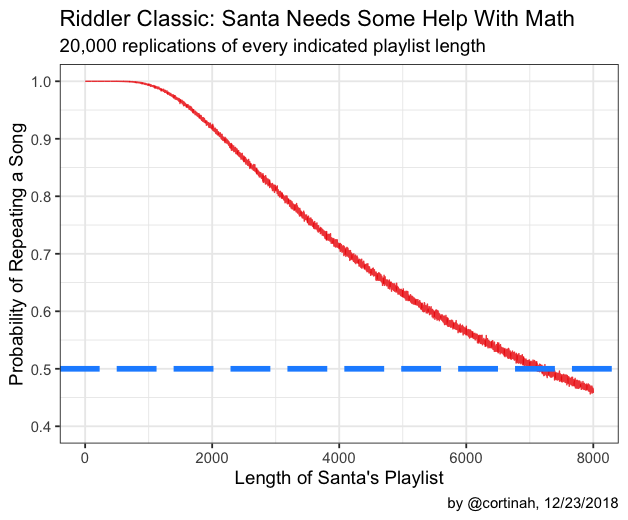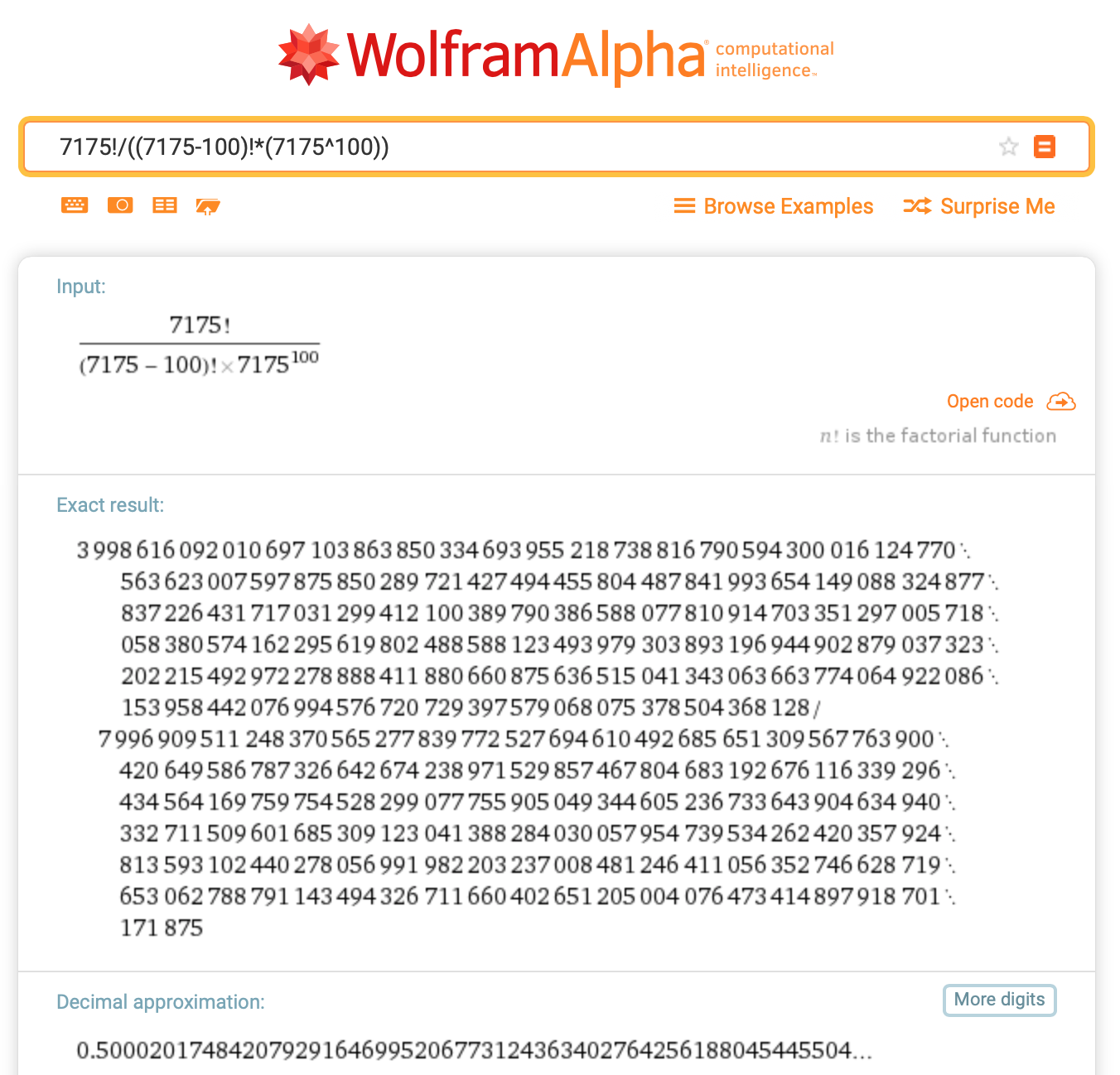4 min read

# The Riddler: Santa Needs Some Help With Math

In the last Riddler of the year I attempt to solve both the Express and Classic Riddlers! Please see below the Express for the solution to the Classic.

# Riddler Express: How Long Will it Take Santa to Place his Reindeer?

We need to find out how long it will take Santa to place his reindeer in the correct sled positions, if he proceeds at random. It takes a minute to harness a reindeer, and a reindeer will grunt to indicate it’s in the right position.

Thinking in terms of a probability tree, if we consider the first position of the sled, the expected time that it will take to place the reindeer correctly looks like this:The expected time that it will take for the first reindeer to be placed correctly is the sum of all the paths leading to the green circles. For example:

$$E[T_{8}] = \left (\frac{1}{8} \cdot 1 \right) + \left (\frac{7}{8} \cdot \frac{1}{7} \cdot 2 \right ) + \left (\frac{7}{8} \cdot \frac{6}{7} \cdot\frac{1}{6} \cdot 3 \right ) + …$$

This process can be repeated for each of the eight reindeer, with the tree getting one branch shorter for each reindeer that has been already correctly placed. The expected time to place all eight reindeer would then be the sum of the expected time for each reindeer i in 8 to 1:

$$E[T] = \sum E[t_{i}]$$

The product of the fractions can be simplified and solved directly. However, to generalize this problem to a sled with N reindeer, I coded the expected time in R as follows:

reindeer <- function(N) {
if (N==2) return(3/2)
if (N==1) return(1)
n <- N
t <- 2
T <- (1/n)
while (n>2) {
T <- T + ( prod(seq(N-1,2)[1:(t-1)]) / prod(seq(N,3)[1:(t-1)]) ) * ((1/(n-1))*t)
t <- t+1
n <- n-1
}
T <- T + ( prod(seq(N-1,2)[1:(t-2)]) / prod(seq(N,3)[1:(t-2)]) ) * ((1/(n))*t)
T
}

sled <- function(N) sum(sapply(1:N, reindeer))

sled(8)


 22

So it should take Santa on average about 22 minutes to get his eight-reindeer sled ready to go. But what if Santa was riding an XL eighteen-reindeer rig? Over an hour and a half of setup time!

sled(18)


 94.5

Extra credit question: What can Santa do to finish faster? Santa could try what I call the “Mastermind” strategy. That is, start by harnessing all eight reindeer at once, check each reindeer’s position, and then repeat until all are correctly placed. While harnessing all eight at once will take eight minutes, this strategy, should, in the worst case, have the same expected time as the above strategy. However, there is a non-zero chance that Santa will randomly place multiple reindeer correctly in every round, thus reducing the overall setup time.

# Riddler Classic: How Long is Santa’s Playlist?

How long is Santa’s music playlist if in a random 100-song sequence the probability of repeating a song is 12 ? The reader may observe that this question is a variation of the classic birthday problem in probability. In this instance, instead of 365 days we have a playlist of unknown length l. Furthermore, instead of a group of n people, we have a playlist of 100 songs. Accordingly, following the birthday problem’s solution, the length of Santa’s playlist l must satisfy:

$$P=\frac{l!}{l^{100}\cdot (l-100)!}=\frac{1}{2} \qquad \qquad (1)$$

An alternative empirical approach to solving this problem is a Monte Carlo simulation of the daily playlist, counting the number of times a song is repeated for a playlists of varying lengths. This will not provide an exact solution, but an approximation of the range. We do this as follows, which produces the chart below, based on 20,000 simulations of playlists of lengths 1 to 8,000:

library(tidyverse)

santa_list <- function(min_list=1, max_list, replications=20000){
search <- function(length=length, replications=20000) {

trial <- function(playlist_length) any(duplicated( sample(1:playlist_length, 100, replace=T) ))

trials <- replicate(replications, trial(length))
sum(trials) / length(trials)
}

data.frame(length=min_list:max_list,
prob=map_dbl(min_list:max_list,~ search(length=.x, replications=replications)))
}

results <- santa_list(max_list=8000)

ggplot(results, aes(x=length, y=prob)) +geom_line(alpha=0.8, color='red2') +
theme_bw(base_size = 14) +
scale_y_continuous(breaks=seq(0.4,1,.1),lim=c(0.4,1)) +
labs(y='Probability of Repeating a Song', x="Length of Santa's Playlist",
title='Riddler Classic: Santa Needs Some Help With Math',
subtitle='20,000 replications of every indicated playlist length',
caption='by @cortinah, 12/23/2018') +
geom_hline(yintercept = 0.5, color='dodgerblue', size=2, linetype=5)Examining the results of the simulation, we notice that the curve crosses the P=12 line near l=7,150. However, this is an approximate value subject to the randomness in our simulation. We can use this value to help us find an exact solution to equation (1) above. Using Wolfram Alpha we quickly find that the closest integer solution with P=12 is 7,175. That is the length of Santa’s playlist.